# ML Aggarwal Class 8 Solutions for ICSE Maths Chapter 13 Understanding Quadrilaterals Check Your Progress

## ML Aggarwal Class 8 Solutions for ICSE Maths Chapter 13 Understanding Quadrilaterals Check Your Progress

Question 1.
From the given diagram, find the value of x.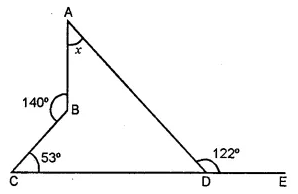Solution:Question 2.
If two angles of a quadrilateral are 77° and 51°, and out of the remaining two angles, one angle is 10° smaller than the other, find these angles.
Solution: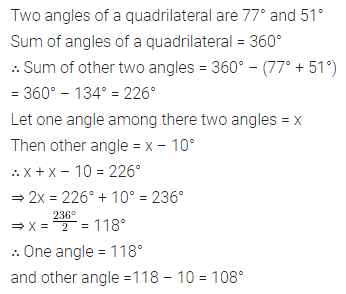Question 3.
In the given figure, AB || DC, ∠A = 74° and ∠B : ∠C = 4 : 5. Find
(i) ∠D
(ii) ∠B
(iii) ∠CSolution: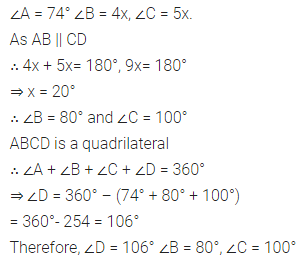Question 4.
In quadrilateral ABCD, ∠A : ∠B : ∠C : ∠D = 3 : 4 : 6 : 7. Find all the angles of the quadrilateral. Hence, prove that AB and DC are parallel. Is BC also parallel to AD?
Solution: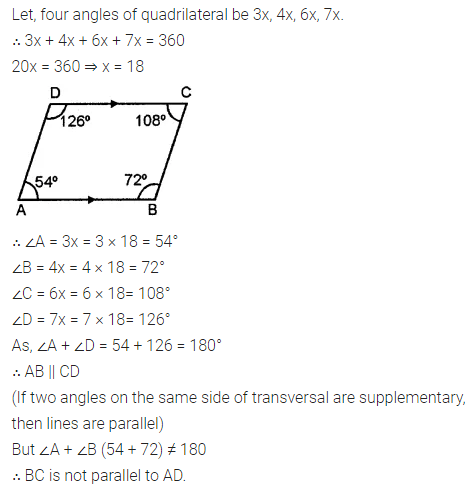Question 5.
One angle of a parallelogram is two-third of the other. Find the angles of the parallelogram.
Solution:Question 6.
In the given figure, ABCD is a kite. If ∠BCD = 52° and ∠ADB = 42°, find the values of x, y, and z.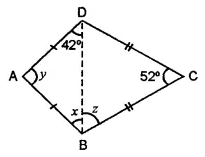Solution: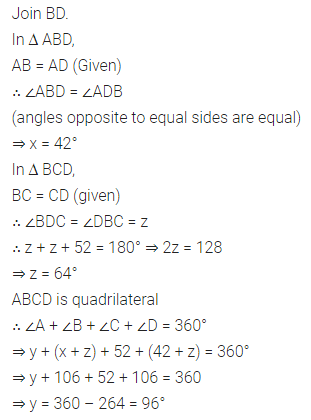Question 7.
In the given figure, ABCD is a rectangle. Prove that AC = BD.Solution: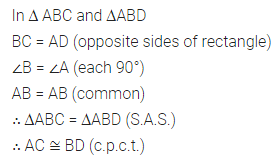Question 8.
In the given figure, ABCD is a rhombus and EDC is an equilateral triangle. If ∠DAB = 48°, find
(i) ∠BEC
(ii) ∠DEB
(iii) ∠BFC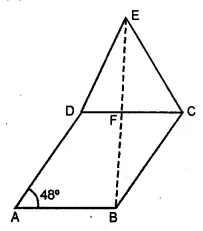Solution:Question 9.
Find the number of sides of a regular polygon if each of its interior angles is 168°.
Solution: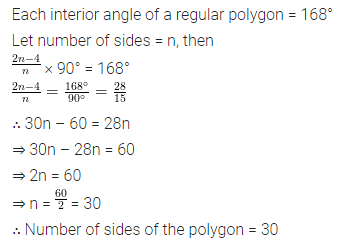Question 10.
If the sum of interior angles of a polygon is 3780° find the number of sides.
Solution:Question 11.
The angles of a hexagon are (2x + 5)°, (3x – 5)°, (x + 40)°, (2x + 20)°, (2x + 25)° and (2x + 35)°. Find the value of x.
Solution:Question 12.
Two angles of a polygon are right angles and every other angle is 120°. Find the number of sides of the polygon.
Solution:Question 13.
The sum of interior angles of a regular polygon is twice the sum of its exterior angles. Find the number of sides of the polygon.
Solution:Question 14.
An exterior angle of a regular polygon is one- fourth of its interior angle. Find the number of sides in the polygon.
Solution: